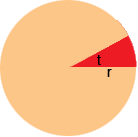Name: Natashia Who is asking: Student Level of the question: Middle Question: How do you find the area of a shaded sector of a circle? Natashia, The area of the sector depends on the radius of the circle r and the measure of the angle t.If the measure of the angle is 360o the the sector is the entire circle and the area isr2area =r2 If the measure of the angle is 180o the the sector is one half the circle and the area is 1/2r2area = 1/2r2 If the measure of the angle is 90o the the sector is one quarter the circle and the area is 1/4r2area = 1/4r2 If the measure of the angle is 45o the the sector is one eighth the circle and the area is 1/8r2area = 1/8r2 What you can see is that the area of the sector is a fraction of the area of the circle , and the fraction is the same fraction that the angle is of 360o. Thus if the radius of the circle is r and the central angle of the sector has measure t then the area of the sector is t/360r2. Penny